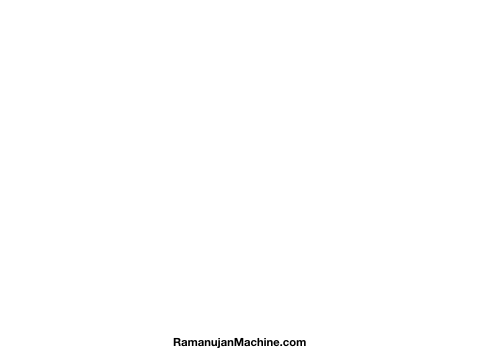# Researchers Develop ‘Ramanujan Machine’ To Discover New Mathematical Formulas

• Ramanujan Machine automatically produces conjectures on fundamental constants.
• It’s not actually a machine, but a combination of algorithms to generate mathematical formulas for fundamental constants.

Fundamental mathematical constants such as e and π play a crucial in various fields of science, ranging from abstract mathematics and geometry to fundamental physics, chemistry, and biology.  However, for centuries new conjectures (mathematical statements that are proposed as true statements) have been scarce and sporadic.

To accelerate the process of discoveries, particularly in the branch of mathematics, researchers at the Technion – Israel Institute of Technology have developed a new concept called the Ramanujan Machine.

It’s not actually a machine, but a network of computers running algorithms to generate mathematical formulas for fundamental constants. For example, it takes a constant (such a π) as input and comes up with an equation containing an infinite series, whose value will be equal to the constant (π).

The derivation of equations isn’t a part of the Ramanujan Machine. Finding the proofs of these equations is something that others (humans) should do.

### Why Is It Named After The Indian Mathematician?

The machine reflects the way Srinivasa Ramanujan worked during his short life. He was a self-taught mathematician who grew up in India. At the age of 26, he moved to England and spent nearly five years in Cambridge, publishing extraordinary mathematical research.

Throughout this life, he compiled over 3,000 identities and equations, including Ramanujan prime, partition formulae, mock theta functions, and Landau–Ramanujan constant.

Instead of working on proofs, Ramanujan obtained results to complex problems and let others formally trained mathematicians find the proofs for them. This is why he was often described as a conjecture machine.

Reference: arXiv:1907.00205 | Wikipedia | GitHub

### How Does It Work?

Israeli researchers have replicated this approach using modern computing power. They developed two algorithms to find conjectures:

1. A variant of the Meet-In-The-Middle algorithm
2. A Gradient Descent optimization algorithm

Since both are based on matching numerical values, they conjecture equations without evaluating any proof. This kind of approach is useful for fundamental constants for which no underlying mathematical structure is known.The algorithm finds conjectures in the form of continued fractions (fractions of infinite length). The machine’s objective is to provide conjectures in the form of mathematical formulas that can be later analyzed and proved by others.

The Ramanujan Machine has already come up with several conjecture formulas by matching numerical values. This includes both well-known and previously unknown continued fraction representations of e and π.

Researchers hope that their machine will inspire future generations of mathematicians and physicists. They have set up a website where users can propose new algorithms or suggest proofs for the conjectures. Anybody can run the Ramanujan Machine to discover new mathematical conjectures, which will be named after the user.Written by
###### Varun Kumar

Varun Kumar is a professional science and technology journalist and a big fan of AI, machines, and space exploration. He received a Master's degree in computer science from GGSIPU University. To find out about his latest projects, feel free to directly email him at [email protected]